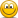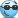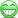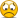# Insane FlickShot & Ace

#### LCp0wer

##### LE
CSGO Pro League
Hello!
I hope everyone will like it)
Sorry for the bad quality! My first video.

Liked the video?=) Click "like"•RoteN and FD^GoD

#### ToX

##### Retired Staff
Eligible for Pro League Beta
Nice, send me ur UMP mirage demo.

•LCp0wer

#### LCp0wer

##### LE
CSGO Pro League
Nice, send me ur UMP mirage demo.
ty.

[Auth details]
;
;
;
; [ESP settings]
wall=0
wall2=0
dlight=0
decay=1
autointensity=0
intensity=4
dteam=1
crosshair=0
esp=2
box=2
boxsize=50
boxmulti=1
visesp=1
listesp=0
dis=0
hp=0
name=0
xesp=0
seq=0
pixel=0
pixelr=85
pixelg=107
pixelb=47
pixela=240
teamesp=0
disp=0
dispx=400
dispy=0
entity=0
noflash=0.10
weapon=0
listx=1750
listy=425
listesp=0
;V=0x56
espkey=0x56
;
; [Aimbot settings]
aimbot=1
index=0
totalbones=5
cleandraw=0
smoothaim=3.00
multibone=0
bone=8
bone1=0
bone2=0
aimheight=0.00
aimright=0.00
fov=2.00
teamaim=1
aimdraw=0
aimkey=4
aimbutton=56
vischeck=1
aimclose=2
cdelta=6.00
shoot=2
rcsfov=10
aimtime=25.00
recoil=0.00
recoilx=0.63
recoilkey=0
recoiltype=4
nohop=1
flickshot=1
flickbutton=4
ammomgmt=1
;
; [Triggerbot]
triggerbot=0
triggerdelay=0
triggerkey=6
triggerfov=0.00
triggerbones=0
triggerdraw=0
triggerbutton=58
triggerbone=0
triggerbone0=0
triggerbone1=1
triggerbone2=2
triggerbone3=3
triggerbone4=4
triggerbone5=5
triggerbone6=6
triggerbone7=8
triggerbone8=35
triggervischeck=2
;
; [MISC]
engcheck=1
neww2s=0
bhop=0
bhopkey=0x43
nofont=1
panickey=0x2E
crosswidth=1
;
; [Weaponconfig system]
weaponconfig=1
weaponaimkey=1
weapontriggercfg=1
weapontriggerkey=1
;
pistol_aimbot=1
pistol_aimkey=5
pistol_fov=1.70
pistol_rcsfov=20
pistol_aimright=0.00
pistol_aimheight=0.00
pistol_smoothaim=17.00
pistol_supersmooth=7
pistol_multibone=0
pistol_bone=8
pistol_bone1=7
pistol_recoil=0.00
pistol_recoilx=0.88
pistol_crosshair=0
pistol_triggerbot=0
pistol_triggerbones=0
pistol_nohop=0
pistol_aimtime=0
pistol_randomspot=0
;
deagle_aimbot=1
deagle_aimkey=5
deagle_fov=1.60
deagle_rcsfov=20
deagle_aimright=0.00
deagle_aimheight=0.00
deagle_smoothaim=2.50
deagle_supersmooth=4
deagle_multibone=0
deagle_bone=8
deagle_bone1=7
deagle_recoil=0.00
deagle_recoilx=0.88
deagle_crosshair=0
deagle_triggerbot=0
deagle_triggerbones=0
deagle_nohop=1
deagle_aimtime=300.00
deagle_randomspot=0
;
sniper_aimbot=1
sniper_aimkey=5
sniper_fov=4.15
sniper_rcsfov=0
sniper_smoothaim=2.50
sniper_supersmooth=4
sniper_multibone=4
sniper_bone=5
sniper_bone1=3
sniper_bone2=6
sniper_bone3=0
sniper_aimheight=0.00
sniper_recoil=0.00
sniper_recoilx=0.00
sniper_crosshair=1
sniper_triggerbot=0
sniper_aimright=0.00
sniper_triggerbones=0
sniper_nohop=1
sniper_aimtime=100.00
sniper_randomspot=3
;
autosniper_aimbot=1
autosniper_aimkey=1
autosniper_fov=2.00
autosniper_rcsfov=20
autosniper_aimright=0.00
autosniper_aimheight=0.00
autosniper_smoothaim=7.00
autosniper_supersmooth=8
autosniper_multibone=2
autosniper_bone=6
autosniper_bone1=5
autosniper_bone2=8
autosniper_recoil=0.85
autosniper_recoilx=0.88
autosniper_crosshair=1
autosniper_triggerbot=2
autosniper_triggerbones=0
autosniper_nohop=1
autosniper_aimtime=0.00
autosniper_randomspot=0
;
rifle_aimbot=1
rifle_aimkey=1
rifle_fov=1.50
rifle_rcsfov=35
rifle_aimright=0.00
rifle_aimheight=0.00
rifle_smoothaim=3.00
rifle_supersmooth=8
rifle_multibone=1
rifle_bone=6
rifle_bone1=8
rifle_recoil=0.60
rifle_recoilx=0.53
rifle_crosshair=0
rifle_triggerbot=0
rifle_triggerbones=0
rifle_nohop=1
rifle_aimtime=1200.00
rifle_randomspot=1
rifle_recoilafter=0
;
smg_aimbot=1
smg_aimkey=1
smg_fov=1.60
smg_rcsfov=30
smg_aimright=0.00
smg_aimheight=0.00
smg_smoothaim=7.00
smg_supersmooth=8
smg_multibone=1
smg_bone=6
smg_bone1=8
smg_recoil=0.65
smg_recoilx=0.50
smg_crosshair=0
smg_triggerbot=0
smg_triggerbones=0
smg_nohop=0
smg_aimtime=1200.00
smg_randomspot=1
;
shotgun_aimbot=1
shotgun_aimkey=4
shotgun_fov=2.00
shotgun_rcsfov=25
shotgun_aimright=0.00
shotgun_aimheight=0.00
shotgun_smoothaim=3.60
shotgun_supersmooth=8
shotgun_multibone=9
shotgun_bone=8
shotgun_bone1=7
shotgun_bone2=6
shotgun_bone3=5
shotgun_bone4=3
shotgun_bone5=96
shotgun_bone6=70
shotgun_bone7=64
shotgun_bone8=36
shotgun_bone9=80
shotgun_recoil=0.00
shotgun_recoilx=0.88
shotgun_crosshair=0
shotgun_triggerbot=0
shotgun_triggerbones=0
shotgun_nohop=1
shotgun_aimtime=200.00
;
pistolhp_aimbot=1
pistolhp_aimkey=4
pistolhp_triggerbot=2
pistolhp_bone=8
pistolhp_bone1=5
pistolhp_bone2=4
pistolhp_bone3=3
pistolhp_bone4=2
pistolhp_smoothaim=7.00
pistolhp_fov=1.75
pistolhp_multibone=0
pistolhp_aimheight=0.00
pistolhp_aimright=0.00
pistolhp_recoil=0.70
pistolhp_recoilx=0.13
pistolhp_triggerbones=0
pistolhp_crosshair=0
pistolhp_supersmooth=8
pistolhp_rcsfov=25
pistolhp_nohop=1
pistolhp_aimtime=0.00
pistolhp_shoot=0
pistolhp_randomspot=0
;
knife_aimbot=0
knife_triggerbot=0
;
; [More]
bone3=0
bone4=2
bone5=3
pistol_bone3=1
pistol_bone4=2
pistol_bone5=3
deagle_bone3=1
deagle_bone4=2
deagle_bone5=3
sniper_bone3=1
sniper_bone4=8
sniper_bone5=3
autosniper_bone3=1
autosniper_bone4=2
autosniper_bone5=3
rifle_bone3=6
rifle_bone4=2
rifle_bone5=3
smg_bone3=1
smg_bone4=2
smg_bone5=3
shotgun_bone3=1
shotgun_bone4=2
shotgun_bone5=3
pistol_shoot=0
deagle_shoot=0
sniper_shoot=2
autosniper_shoot=0
rifle_shoot=0
smg_shoot=0
shotgun_shoot=2
shotgun_randomspot=0
bhopmaxdelay=20
bhopmindelay=10
supersmooth=5
randomspot=0
closefov=0.00
closefovdist=0
recoilafter=0
triggerrandomdelay=0
triggermindelay=0
ignorejumping=0
pistol_closefov=4.50
pistol_closefovdist=3
pistol_aimonshoot=0
pistol_closefovscoped=0
pistol_recoilafter=0
pistol_triggerdelay=0
pistol_triggerkey=5
pistol_triggerrandomdelay=0
pistol_triggermindelay=0
pistol_tfov=1.70
pistol_triggerbone0=14
pistol_triggerbone1=5
pistol_triggerbone2=5
pistol_triggerbone3=5
pistol_triggerbone4=5
pistol_triggerbone5=5
pistol_triggerbone6=5
pistol_triggerbone7=5
pistol_triggerbone8=5
pistolhp_closefov=4.50
pistolhp_closefovdist=3
pistolhp_aimonshoot=0
pistolhp_closefovscoped=0
pistolhp_recoilafter=0
pistolhp_triggerdelay=0
pistolhp_triggerkey=4
pistolhp_triggerrandomdelay=0
pistolhp_triggermindelay=0
pistolhp_tfov=2.50
pistolhp_triggerbone0=14
pistolhp_triggerbone1=6
pistolhp_triggerbone2=4
pistolhp_triggerbone3=0
pistolhp_triggerbone4=1
pistolhp_triggerbone5=2
pistolhp_triggerbone6=3
pistolhp_triggerbone7=8
pistolhp_triggerbone8=35
deagle_closefov=6.00
deagle_closefovdist=4
deagle_aimonshoot=0
deagle_closefovscoped=0
deagle_recoilafter=0
deagle_triggerdelay=0
deagle_triggerkey=5
deagle_triggerrandomdelay=0
deagle_triggermindelay=0
deagle_tfov=1.50
deagle_triggerbone0=14
deagle_triggerbone1=5
deagle_triggerbone2=5
deagle_triggerbone3=5
deagle_triggerbone4=5
deagle_triggerbone5=5
deagle_triggerbone6=5
deagle_triggerbone7=5
deagle_triggerbone8=5
sniper_closefov=14.00
sniper_closefovdist=12
sniper_aimonshoot=0
sniper_closefovscoped=10
sniper_recoilafter=0
sniper_triggerdelay=0
sniper_triggerkey=0
sniper_triggerrandomdelay=0
sniper_triggermindelay=0
sniper_tfov=1.50
sniper_triggerbone0=0
sniper_triggerbone1=0
sniper_triggerbone2=0
sniper_triggerbone3=0
sniper_triggerbone4=0
sniper_triggerbone5=0
sniper_triggerbone6=0
sniper_triggerbone7=0
sniper_triggerbone8=0
autosniper_closefov=4.00
autosniper_closefovdist=7
autosniper_aimonshoot=0
autosniper_closefovscoped=5
autosniper_recoilafter=0
autosniper_triggerdelay=0
autosniper_triggerkey=5
autosniper_triggerrandomdelay=0
autosniper_triggermindelay=0
autosniper_tfov=1.50
autosniper_triggerbone0=1
autosniper_triggerbone1=1
autosniper_triggerbone2=2
autosniper_triggerbone3=3
autosniper_triggerbone4=4
autosniper_triggerbone5=5
autosniper_triggerbone6=6
autosniper_triggerbone7=7
autosniper_triggerbone8=8
rifle_closefov=5.00
rifle_closefovdist=12
rifle_aimonshoot=0
rifle_closefovscoped=0
rifle_triggerdelay=0
rifle_triggerkey=5
rifle_triggerrandomdelay=0
rifle_triggermindelay=10
rifle_tfov=2.50
rifle_triggerbone0=14
rifle_triggerbone1=1
rifle_triggerbone2=6
rifle_triggerbone3=0
rifle_triggerbone4=1
rifle_triggerbone5=2
rifle_triggerbone6=3
rifle_triggerbone7=8
rifle_triggerbone8=35
smg_closefov=4.00
smg_closefovdist=1
smg_aimonshoot=0
smg_closefovscoped=0
smg_recoilafter=0
smg_triggerdelay=0
smg_triggerkey=5
smg_triggerrandomdelay=0
smg_triggermindelay=0
smg_tfov=1.50
smg_triggerbone0=14
smg_triggerbone1=5
smg_triggerbone2=5
smg_triggerbone3=5
smg_triggerbone4=5
smg_triggerbone5=5
smg_triggerbone6=5
smg_triggerbone7=5
smg_triggerbone8=5
shotgun_closefov=17.50
shotgun_closefovdist=15
shotgun_aimonshoot=0
shotgun_closefovscoped=0
shotgun_recoilafter=0
shotgun_triggerdelay=0
shotgun_triggerkey=0
shotgun_triggerrandomdelay=0
shotgun_triggermindelay=0
shotgun_tfov=2.50
shotgun_triggerbone0=14
shotgun_triggerbone1=5
shotgun_triggerbone2=5
shotgun_triggerbone3=5
shotgun_triggerbone4=5
shotgun_triggerbone5=5
shotgun_triggerbone6=5
shotgun_triggerbone7=5
shotgun_triggerbone8=5
knife_aimkey=0
aimonshoot=0
closefovscoped=0

•Joao Pacheco

#### Joao Pacheco

##### League Player
ty.

[Auth details]
;
;
;
; [ESP settings]
wall=0
wall2=0
dlight=0
decay=1
autointensity=0
intensity=4
dteam=1
crosshair=0
esp=2
box=2
boxsize=50
boxmulti=1
visesp=1
listesp=0
dis=0
hp=0
name=0
xesp=0
seq=0
pixel=0
pixelr=85
pixelg=107
pixelb=47
pixela=240
teamesp=0
disp=0
dispx=400
dispy=0
entity=0
noflash=0.10
weapon=0
listx=1750
listy=425
listesp=0
;V=0x56
espkey=0x56
;
; [Aimbot settings]
aimbot=1
index=0
totalbones=5
cleandraw=0
smoothaim=3.00
multibone=0
bone=8
bone1=0
bone2=0
aimheight=0.00
aimright=0.00
fov=2.00
teamaim=1
aimdraw=0
aimkey=4
aimbutton=56
vischeck=1
aimclose=2
cdelta=6.00
shoot=2
rcsfov=10
aimtime=25.00
recoil=0.00
recoilx=0.63
recoilkey=0
recoiltype=4
nohop=1
flickshot=1
flickbutton=4
ammomgmt=1
;
; [Triggerbot]
triggerbot=0
triggerdelay=0
triggerkey=6
triggerfov=0.00
triggerbones=0
triggerdraw=0
triggerbutton=58
triggerbone=0
triggerbone0=0
triggerbone1=1
triggerbone2=2
triggerbone3=3
triggerbone4=4
triggerbone5=5
triggerbone6=6
triggerbone7=8
triggerbone8=35
triggervischeck=2
;
; [MISC]
engcheck=1
neww2s=0
bhop=0
bhopkey=0x43
nofont=1
panickey=0x2E
crosswidth=1
;
; [Weaponconfig system]
weaponconfig=1
weaponaimkey=1
weapontriggercfg=1
weapontriggerkey=1
;
pistol_aimbot=1
pistol_aimkey=5
pistol_fov=1.70
pistol_rcsfov=20
pistol_aimright=0.00
pistol_aimheight=0.00
pistol_smoothaim=17.00
pistol_supersmooth=7
pistol_multibone=0
pistol_bone=8
pistol_bone1=7
pistol_recoil=0.00
pistol_recoilx=0.88
pistol_crosshair=0
pistol_triggerbot=0
pistol_triggerbones=0
pistol_nohop=0
pistol_aimtime=0
pistol_randomspot=0
;
deagle_aimbot=1
deagle_aimkey=5
deagle_fov=1.60
deagle_rcsfov=20
deagle_aimright=0.00
deagle_aimheight=0.00
deagle_smoothaim=2.50
deagle_supersmooth=4
deagle_multibone=0
deagle_bone=8
deagle_bone1=7
deagle_recoil=0.00
deagle_recoilx=0.88
deagle_crosshair=0
deagle_triggerbot=0
deagle_triggerbones=0
deagle_nohop=1
deagle_aimtime=300.00
deagle_randomspot=0
;
sniper_aimbot=1
sniper_aimkey=5
sniper_fov=4.15
sniper_rcsfov=0
sniper_smoothaim=2.50
sniper_supersmooth=4
sniper_multibone=4
sniper_bone=5
sniper_bone1=3
sniper_bone2=6
sniper_bone3=0
sniper_aimheight=0.00
sniper_recoil=0.00
sniper_recoilx=0.00
sniper_crosshair=1
sniper_triggerbot=0
sniper_aimright=0.00
sniper_triggerbones=0
sniper_nohop=1
sniper_aimtime=100.00
sniper_randomspot=3
;
autosniper_aimbot=1
autosniper_aimkey=1
autosniper_fov=2.00
autosniper_rcsfov=20
autosniper_aimright=0.00
autosniper_aimheight=0.00
autosniper_smoothaim=7.00
autosniper_supersmooth=8
autosniper_multibone=2
autosniper_bone=6
autosniper_bone1=5
autosniper_bone2=8
autosniper_recoil=0.85
autosniper_recoilx=0.88
autosniper_crosshair=1
autosniper_triggerbot=2
autosniper_triggerbones=0
autosniper_nohop=1
autosniper_aimtime=0.00
autosniper_randomspot=0
;
rifle_aimbot=1
rifle_aimkey=1
rifle_fov=1.50
rifle_rcsfov=35
rifle_aimright=0.00
rifle_aimheight=0.00
rifle_smoothaim=3.00
rifle_supersmooth=8
rifle_multibone=1
rifle_bone=6
rifle_bone1=8
rifle_recoil=0.60
rifle_recoilx=0.53
rifle_crosshair=0
rifle_triggerbot=0
rifle_triggerbones=0
rifle_nohop=1
rifle_aimtime=1200.00
rifle_randomspot=1
rifle_recoilafter=0
;
smg_aimbot=1
smg_aimkey=1
smg_fov=1.60
smg_rcsfov=30
smg_aimright=0.00
smg_aimheight=0.00
smg_smoothaim=7.00
smg_supersmooth=8
smg_multibone=1
smg_bone=6
smg_bone1=8
smg_recoil=0.65
smg_recoilx=0.50
smg_crosshair=0
smg_triggerbot=0
smg_triggerbones=0
smg_nohop=0
smg_aimtime=1200.00
smg_randomspot=1
;
shotgun_aimbot=1
shotgun_aimkey=4
shotgun_fov=2.00
shotgun_rcsfov=25
shotgun_aimright=0.00
shotgun_aimheight=0.00
shotgun_smoothaim=3.60
shotgun_supersmooth=8
shotgun_multibone=9
shotgun_bone=8
shotgun_bone1=7
shotgun_bone2=6
shotgun_bone3=5
shotgun_bone4=3
shotgun_bone5=96
shotgun_bone6=70
shotgun_bone7=64
shotgun_bone8=36
shotgun_bone9=80
shotgun_recoil=0.00
shotgun_recoilx=0.88
shotgun_crosshair=0
shotgun_triggerbot=0
shotgun_triggerbones=0
shotgun_nohop=1
shotgun_aimtime=200.00
;
pistolhp_aimbot=1
pistolhp_aimkey=4
pistolhp_triggerbot=2
pistolhp_bone=8
pistolhp_bone1=5
pistolhp_bone2=4
pistolhp_bone3=3
pistolhp_bone4=2
pistolhp_smoothaim=7.00
pistolhp_fov=1.75
pistolhp_multibone=0
pistolhp_aimheight=0.00
pistolhp_aimright=0.00
pistolhp_recoil=0.70
pistolhp_recoilx=0.13
pistolhp_triggerbones=0
pistolhp_crosshair=0
pistolhp_supersmooth=8
pistolhp_rcsfov=25
pistolhp_nohop=1
pistolhp_aimtime=0.00
pistolhp_shoot=0
pistolhp_randomspot=0
;
knife_aimbot=0
knife_triggerbot=0
;
; [More]
bone3=0
bone4=2
bone5=3
pistol_bone3=1
pistol_bone4=2
pistol_bone5=3
deagle_bone3=1
deagle_bone4=2
deagle_bone5=3
sniper_bone3=1
sniper_bone4=8
sniper_bone5=3
autosniper_bone3=1
autosniper_bone4=2
autosniper_bone5=3
rifle_bone3=6
rifle_bone4=2
rifle_bone5=3
smg_bone3=1
smg_bone4=2
smg_bone5=3
shotgun_bone3=1
shotgun_bone4=2
shotgun_bone5=3
pistol_shoot=0
deagle_shoot=0
sniper_shoot=2
autosniper_shoot=0
rifle_shoot=0
smg_shoot=0
shotgun_shoot=2
shotgun_randomspot=0
bhopmaxdelay=20
bhopmindelay=10
supersmooth=5
randomspot=0
closefov=0.00
closefovdist=0
recoilafter=0
triggerrandomdelay=0
triggermindelay=0
ignorejumping=0
pistol_closefov=4.50
pistol_closefovdist=3
pistol_aimonshoot=0
pistol_closefovscoped=0
pistol_recoilafter=0
pistol_triggerdelay=0
pistol_triggerkey=5
pistol_triggerrandomdelay=0
pistol_triggermindelay=0
pistol_tfov=1.70
pistol_triggerbone0=14
pistol_triggerbone1=5
pistol_triggerbone2=5
pistol_triggerbone3=5
pistol_triggerbone4=5
pistol_triggerbone5=5
pistol_triggerbone6=5
pistol_triggerbone7=5
pistol_triggerbone8=5
pistolhp_closefov=4.50
pistolhp_closefovdist=3
pistolhp_aimonshoot=0
pistolhp_closefovscoped=0
pistolhp_recoilafter=0
pistolhp_triggerdelay=0
pistolhp_triggerkey=4
pistolhp_triggerrandomdelay=0
pistolhp_triggermindelay=0
pistolhp_tfov=2.50
pistolhp_triggerbone0=14
pistolhp_triggerbone1=6
pistolhp_triggerbone2=4
pistolhp_triggerbone3=0
pistolhp_triggerbone4=1
pistolhp_triggerbone5=2
pistolhp_triggerbone6=3
pistolhp_triggerbone7=8
pistolhp_triggerbone8=35
deagle_closefov=6.00
deagle_closefovdist=4
deagle_aimonshoot=0
deagle_closefovscoped=0
deagle_recoilafter=0
deagle_triggerdelay=0
deagle_triggerkey=5
deagle_triggerrandomdelay=0
deagle_triggermindelay=0
deagle_tfov=1.50
deagle_triggerbone0=14
deagle_triggerbone1=5
deagle_triggerbone2=5
deagle_triggerbone3=5
deagle_triggerbone4=5
deagle_triggerbone5=5
deagle_triggerbone6=5
deagle_triggerbone7=5
deagle_triggerbone8=5
sniper_closefov=14.00
sniper_closefovdist=12
sniper_aimonshoot=0
sniper_closefovscoped=10
sniper_recoilafter=0
sniper_triggerdelay=0
sniper_triggerkey=0
sniper_triggerrandomdelay=0
sniper_triggermindelay=0
sniper_tfov=1.50
sniper_triggerbone0=0
sniper_triggerbone1=0
sniper_triggerbone2=0
sniper_triggerbone3=0
sniper_triggerbone4=0
sniper_triggerbone5=0
sniper_triggerbone6=0
sniper_triggerbone7=0
sniper_triggerbone8=0
autosniper_closefov=4.00
autosniper_closefovdist=7
autosniper_aimonshoot=0
autosniper_closefovscoped=5
autosniper_recoilafter=0
autosniper_triggerdelay=0
autosniper_triggerkey=5
autosniper_triggerrandomdelay=0
autosniper_triggermindelay=0
autosniper_tfov=1.50
autosniper_triggerbone0=1
autosniper_triggerbone1=1
autosniper_triggerbone2=2
autosniper_triggerbone3=3
autosniper_triggerbone4=4
autosniper_triggerbone5=5
autosniper_triggerbone6=6
autosniper_triggerbone7=7
autosniper_triggerbone8=8
rifle_closefov=5.00
rifle_closefovdist=12
rifle_aimonshoot=0
rifle_closefovscoped=0
rifle_triggerdelay=0
rifle_triggerkey=5
rifle_triggerrandomdelay=0
rifle_triggermindelay=10
rifle_tfov=2.50
rifle_triggerbone0=14
rifle_triggerbone1=1
rifle_triggerbone2=6
rifle_triggerbone3=0
rifle_triggerbone4=1
rifle_triggerbone5=2
rifle_triggerbone6=3
rifle_triggerbone7=8
rifle_triggerbone8=35
smg_closefov=4.00
smg_closefovdist=1
smg_aimonshoot=0
smg_closefovscoped=0
smg_recoilafter=0
smg_triggerdelay=0
smg_triggerkey=5
smg_triggerrandomdelay=0
smg_triggermindelay=0
smg_tfov=1.50
smg_triggerbone0=14
smg_triggerbone1=5
smg_triggerbone2=5
smg_triggerbone3=5
smg_triggerbone4=5
smg_triggerbone5=5
smg_triggerbone6=5
smg_triggerbone7=5
smg_triggerbone8=5
shotgun_closefov=17.50
shotgun_closefovdist=15
shotgun_aimonshoot=0
shotgun_closefovscoped=0
shotgun_recoilafter=0
shotgun_triggerdelay=0
shotgun_triggerkey=0
shotgun_triggerrandomdelay=0
shotgun_triggermindelay=0
shotgun_tfov=2.50
shotgun_triggerbone0=14
shotgun_triggerbone1=5
shotgun_triggerbone2=5
shotgun_triggerbone3=5
shotgun_triggerbone4=5
shotgun_triggerbone5=5
shotgun_triggerbone6=5
shotgun_triggerbone7=5
shotgun_triggerbone8=5
knife_aimkey=0
aimonshoot=0
closefovscoped=0
1024x768 streched mc off 3.0 sens right?

#### Pedro Moreira

##### Gold Nova II
Nice video bro, is it in matchmaking or faceit?#### quicklyz

##### Newbie
Nice flicks#### Nathanha17

##### Newbie
Nice video, do you use triggerbot or manually shoot with your awp?

#### Heraxion

##### Global Elite
Nice man sick#### haakon

##### Master Guardian II
Eligible for Pro League Beta
Nice#### randomplayer

##### one three three seven
So much power on that USP bro#### FD^GoD

##### Ex Helper
Nice frags, but 1.05 your crosshair in USP lock and skip on the head as fuck#### LCp0wer

##### LE
CSGO Pro League
1024x768 streched mc off 3.0 sens right?
Yeah!
Nice video, do you use triggerbot or manually shoot with your awp?
Not use=)
Nice video bro, is it in matchmaking or faceit?It's not Faceit/MM=) Its Pracc=)

Last edited:

#### ToX

##### Retired Staff
Eligible for Pro League Beta
Still waiting for your PM with demo..